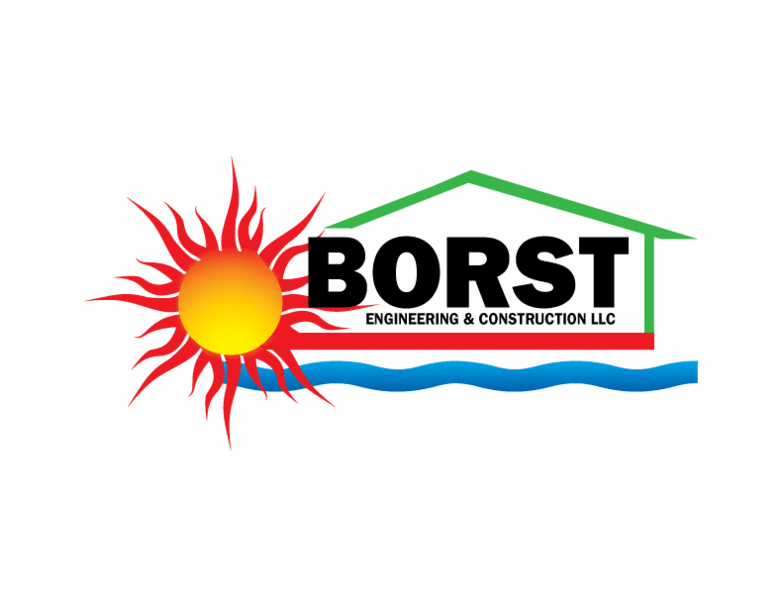Calculator Instructions
Passive Solar Thermal Mass Performance Calculator - Borst Engineering & Construction LLC
 Design Indoor Dry Bulb Temp (Deg F) Daily Irradiance Heat Gain (BTU/Day, from Passive Solar Heat Gain Calculator) Average Daily Irradiance Time Period (Hours/Day, from Passive Solar Heat Gain Calculator) Convection Heat Transfer Coefficient (BTU/Deg F-H-SF, Still Air is 1.56) Thermal Mass Temp prior to Irradiance (Deg F) Thermal Mass Area (SF) Thermal Mass Thickness (Inches, 4-6 typical) Thermal Mass Density (PCF, Concrete is 150) Thermal Mass Specific Heat Capacity (BTU/Deg F-Lb, Concrete is 0.18) Thermal Mass Insulation R-value (Deg F-H-SF/BTU, 2 Inch Thick EPS/XPS is 10) Thermal Mass Absorptivity (%, Concrete is 60-80) Thermal Mass Emissivity (%, Concrete is 85-95)